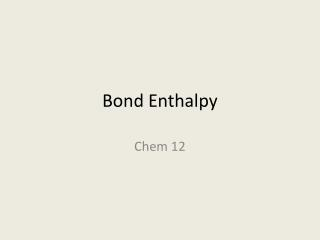DownloadDownload PresentationBond Enthalpy

# Bond Enthalpy

Download Presentation## Bond Enthalpy

- - - - - - - - - - - - - - - - - - - - - - - - - - - E N D - - - - - - - - - - - - - - - - - - - - - - - - - - -
##### Presentation Transcript

1. Bond Enthalpy Chem 12

2. DH0 = 436.4 kJ H2 (g) H (g) + H (g) DH0 = 242.7 kJ Cl2 (g) Cl (g) + Cl (g) DH0 = 431.9 kJ HCl (g) H (g) + Cl (g) DH0 = 498.7 kJ O2 (g) O (g) + O (g) O DH0 = 941.4 kJ N2 (g) N (g) + N (g) O Bond Energies Single bond < Double bond < Triple bond N N The enthalpy change required to break a particular bond in one mole of gaseous molecules is the bond energy. Bond Energy 9.10

3. DH0 = 502 kJ H2O (g) H (g) + OH (g) DH0 = 427 kJ OH (g) H (g) + O (g) = 464 kJ Average OH bond energy = 502 + 427 2 Average bond energy in polyatomic molecules 9.10

4. Bond Energies (BE) and Enthalpy changes in reactions Imagine reaction proceeding by breaking all bonds in the reactants and then using the gaseous atoms to form all the bonds in the products. DH0 = total energy input – total energy released = SBE(reactants) – SBE(products) 9.10

5. 2H2 (g) + O2 (g) 2H2O (g) H2 (g) + Cl2 (g) 2HCl (g) 9.10

6. Use bond energies to calculate the enthalpy change for: H2 (g) + F2 (g) 2HF (g) Type of bonds broken Number of bonds broken Bond energy (kJ/mol) Energy change (kJ) 1 436.4 436.4 1 156.9 156.9 Type of bonds formed Number of bonds formed Bond energy (kJ/mol) Energy change (kJ) H H F H F F 2 568.2 1136.4 DH0 = SBE(reactants) – SBE(products) DH0 = 436.4 + 156.9 – 2 x 568.2 = -543.1 kJ 9.10